# ISC Class 12 Chemistry Question Paper Solution 2019

The ISC Class 12 Chemistry – Paper I (Theory) was conducted on 1st March 2019. The exam started at 2 PM, and students were allotted 3 hours of time duration to finish the paper. Here, we have provided the ISC Class 12 Chemistry Question Paper Solution 2019 for students exam preparation. They can download, both the ISC Class 12 Chemistry Question Paper and Solution pdf 2019 from the link below.

Students can also access the Solved ISC Class 12 Chemistry Previous Year Question Papers compiled at one place. They can have a look at the ISC Class 12 Chemistry Question Paper Solution 2019 below.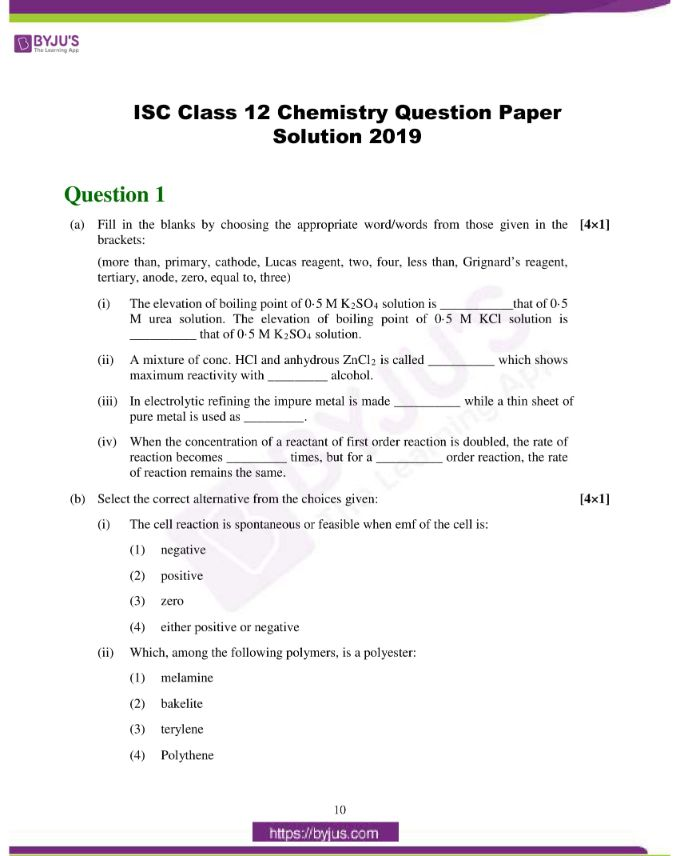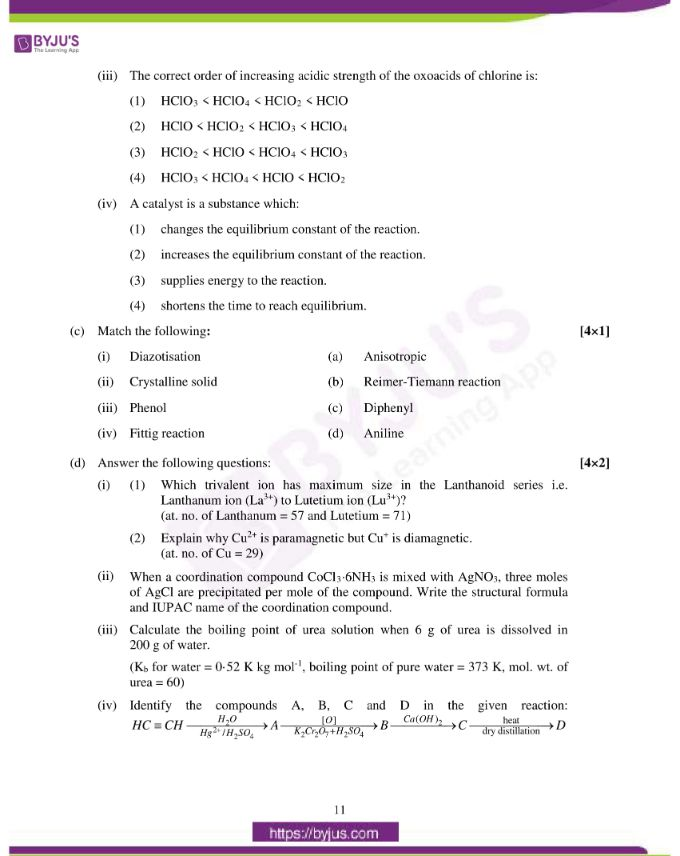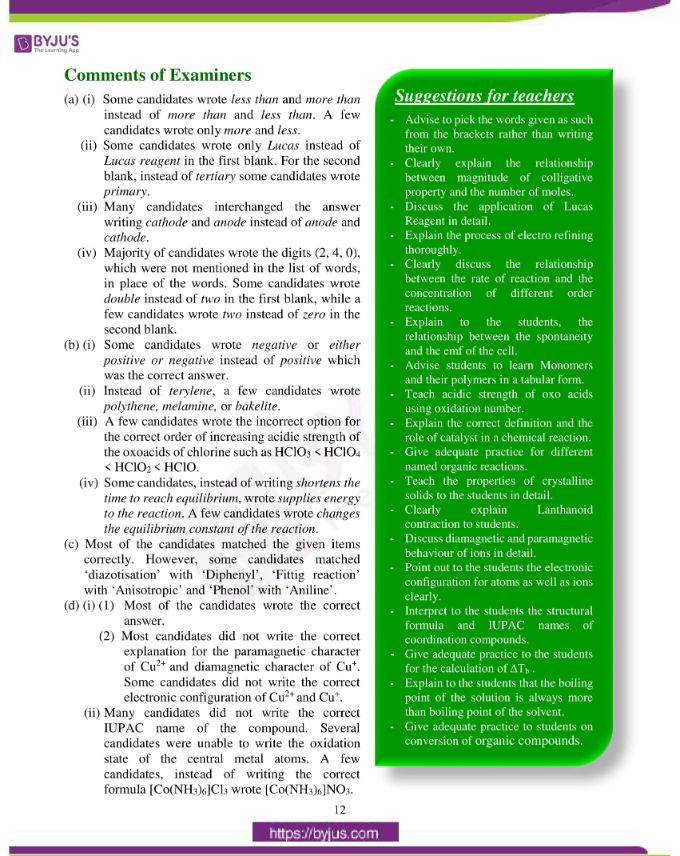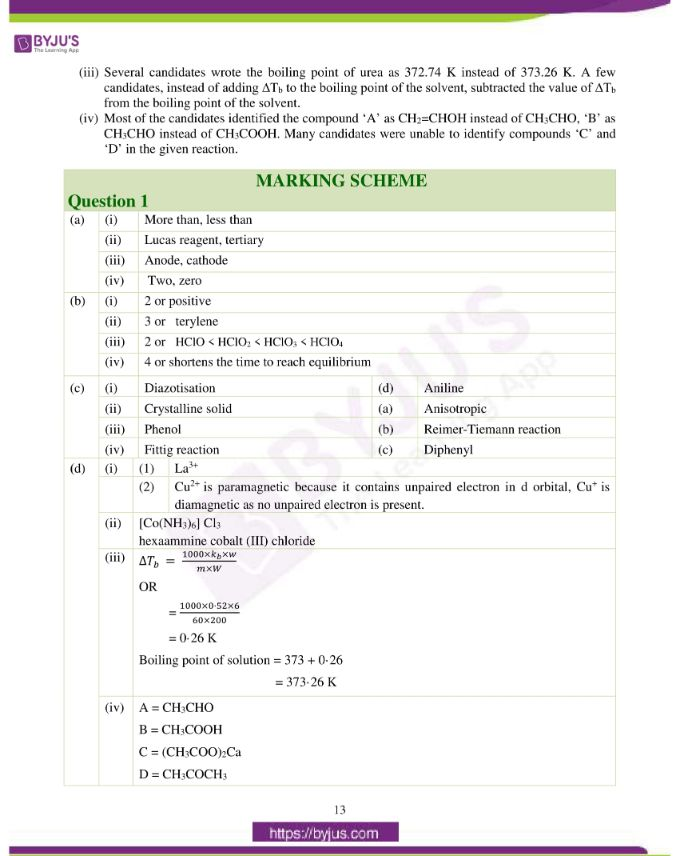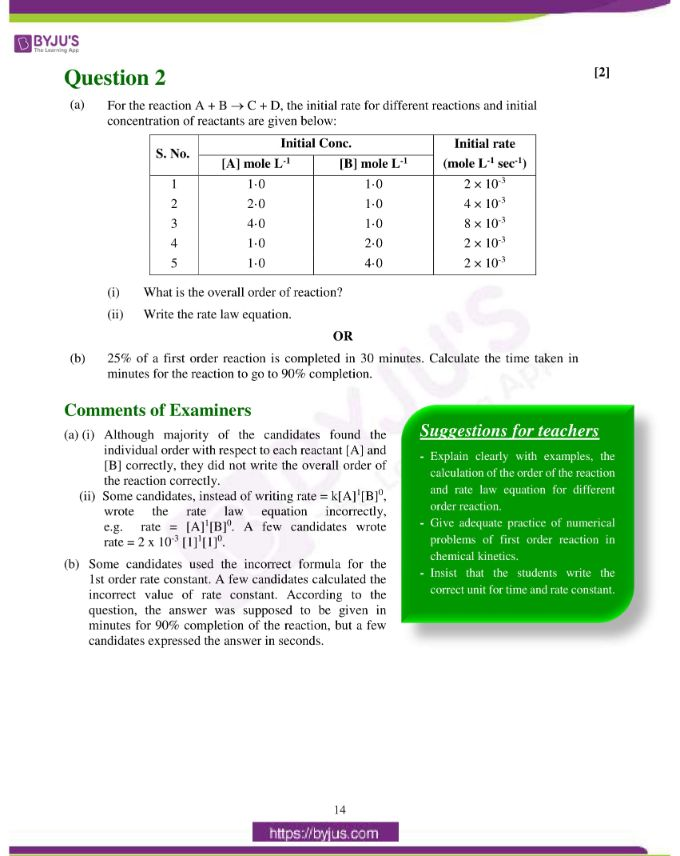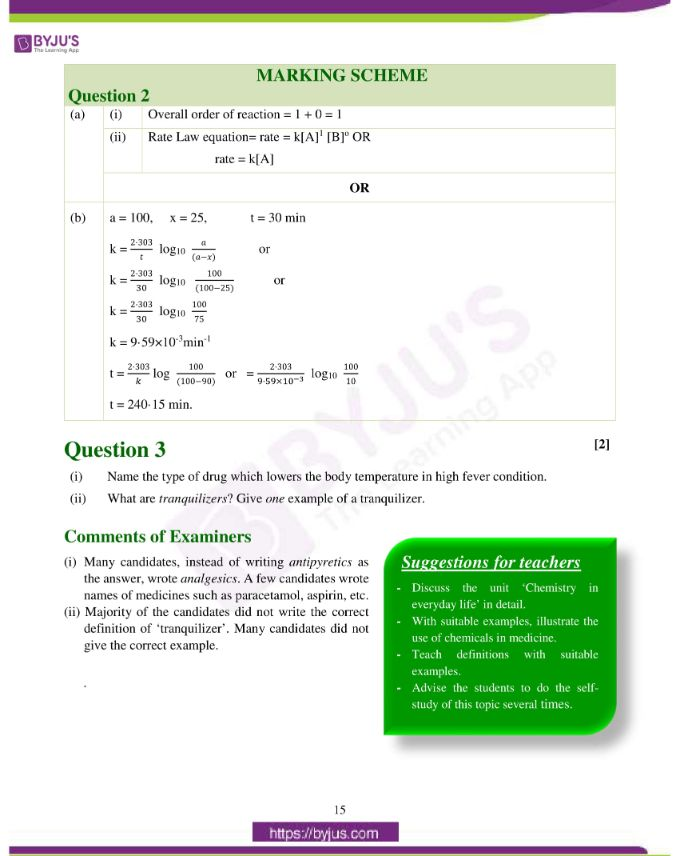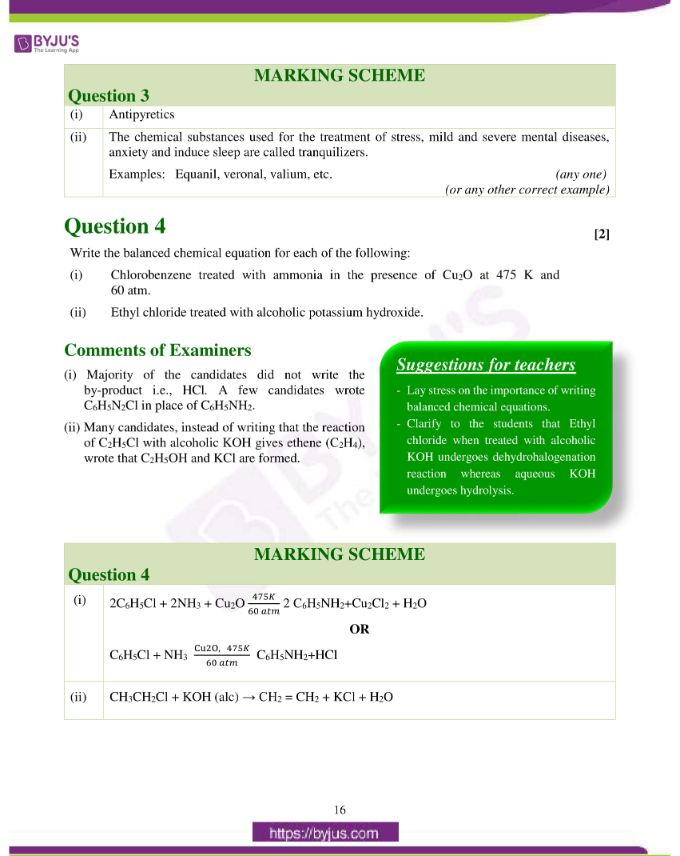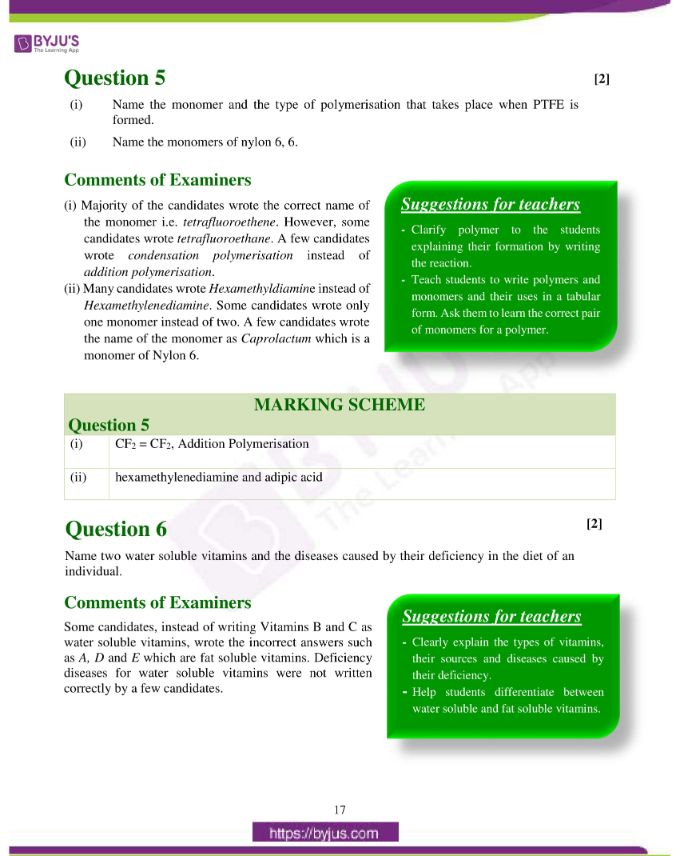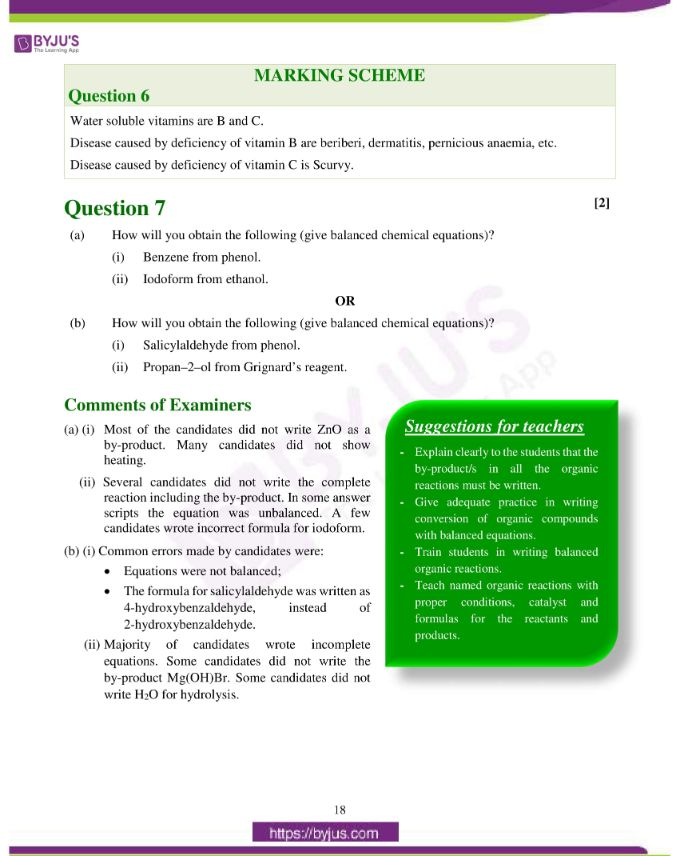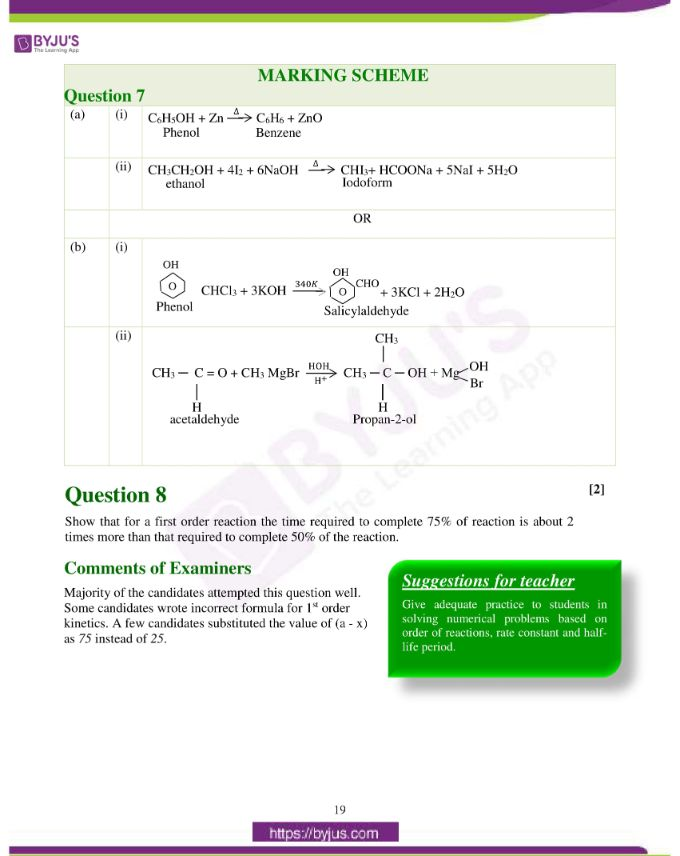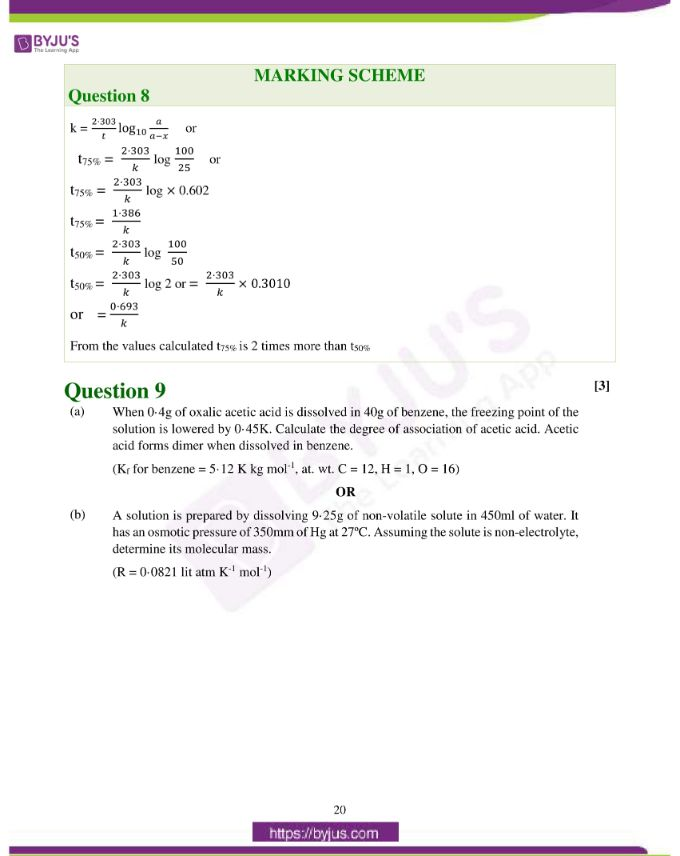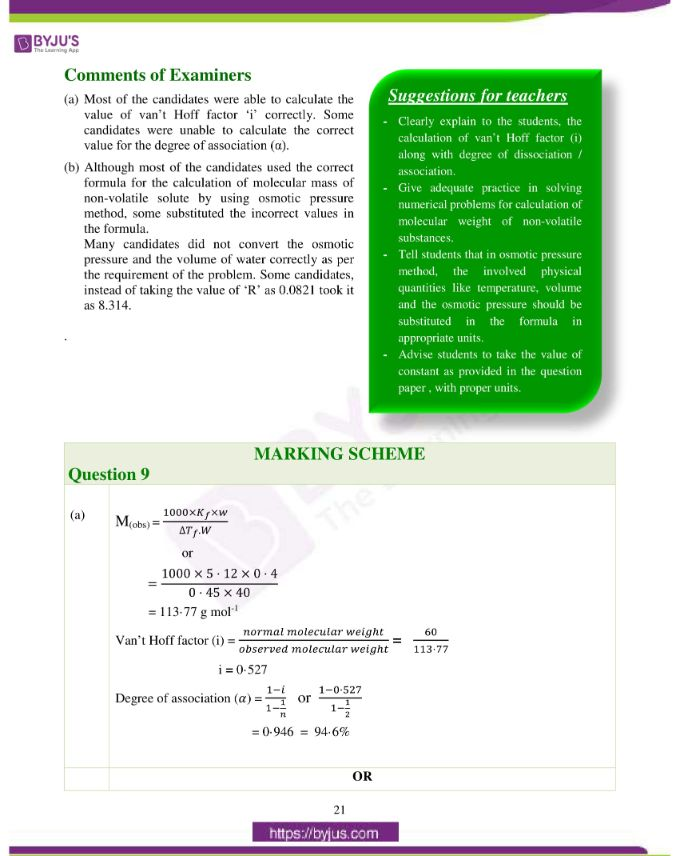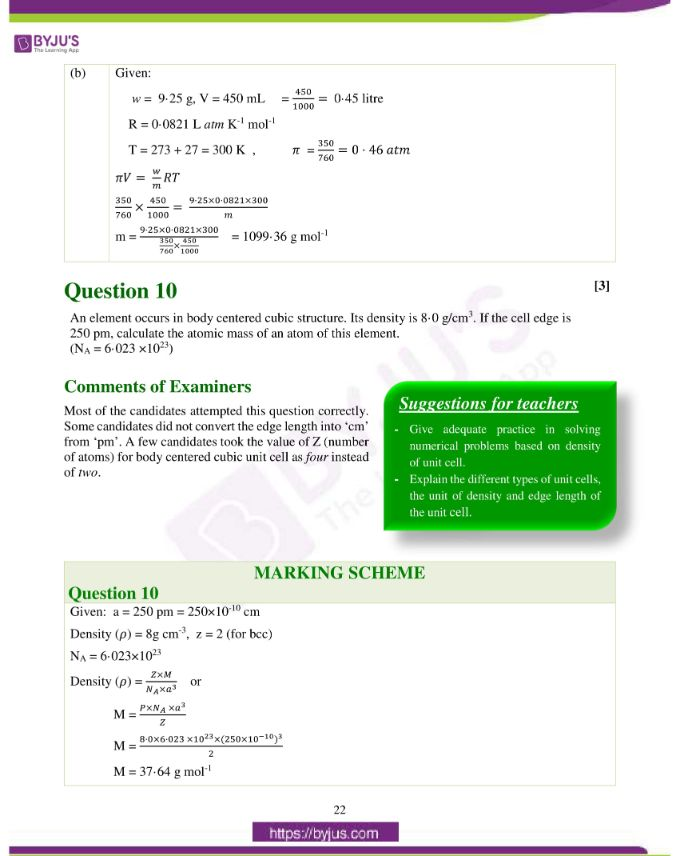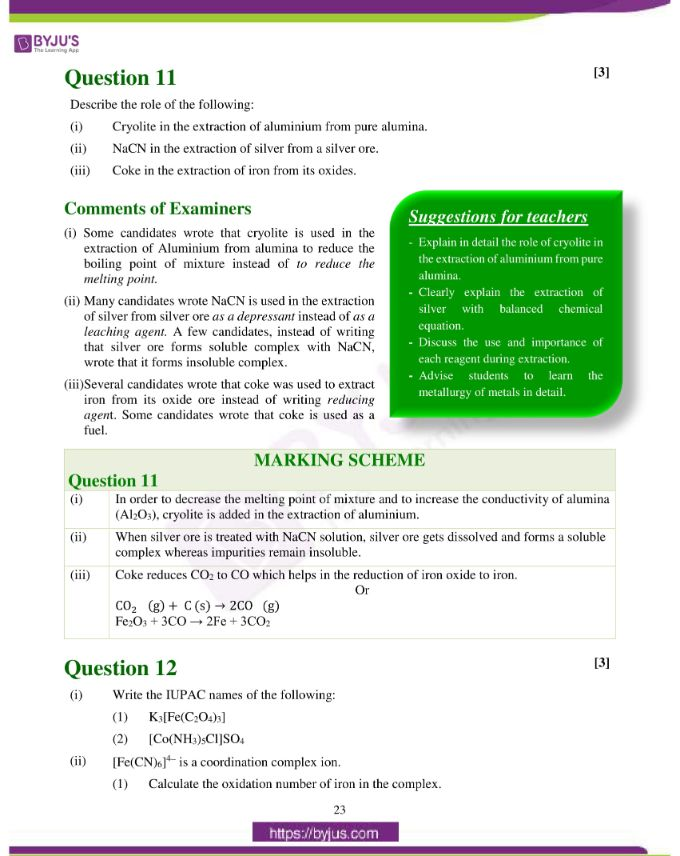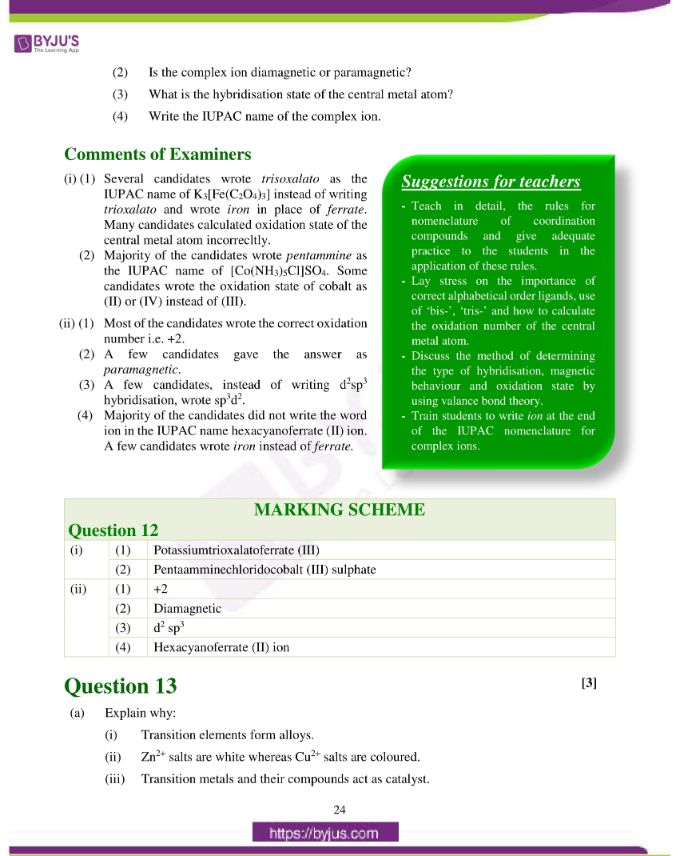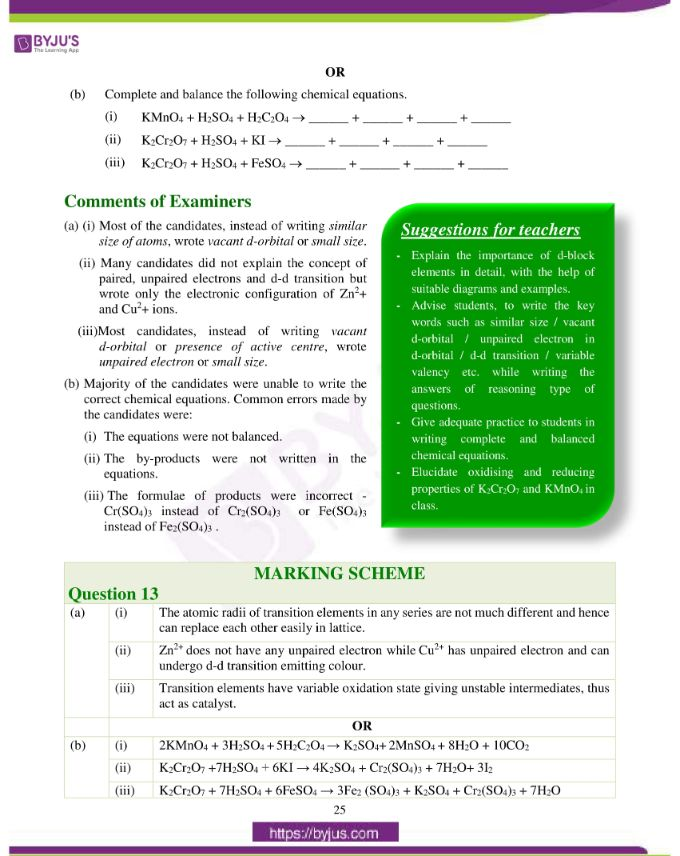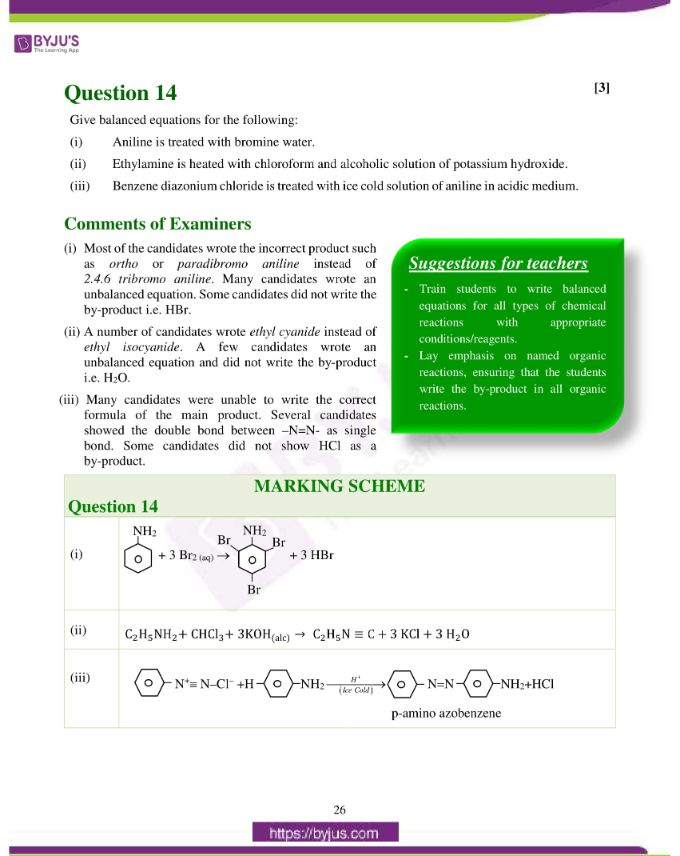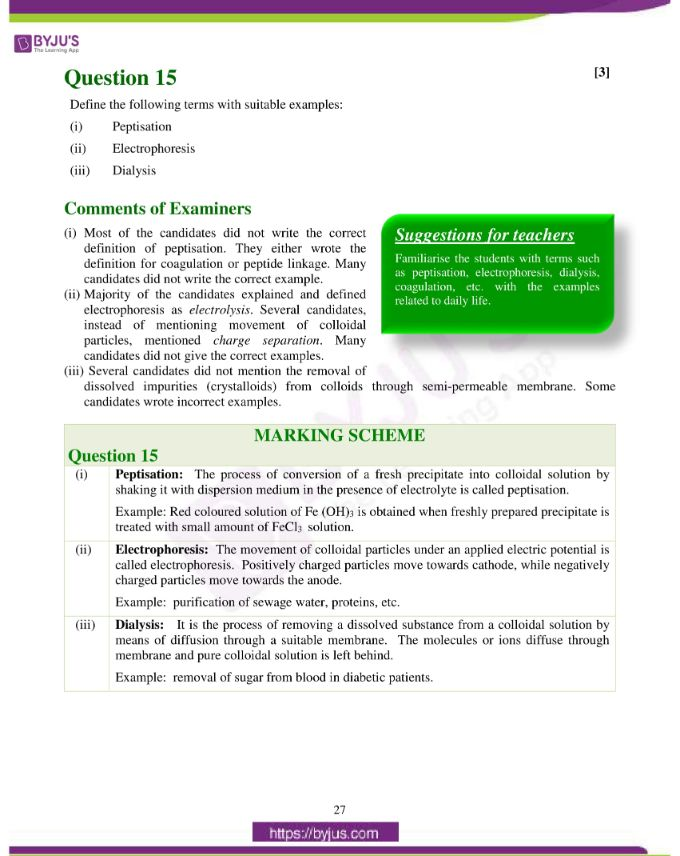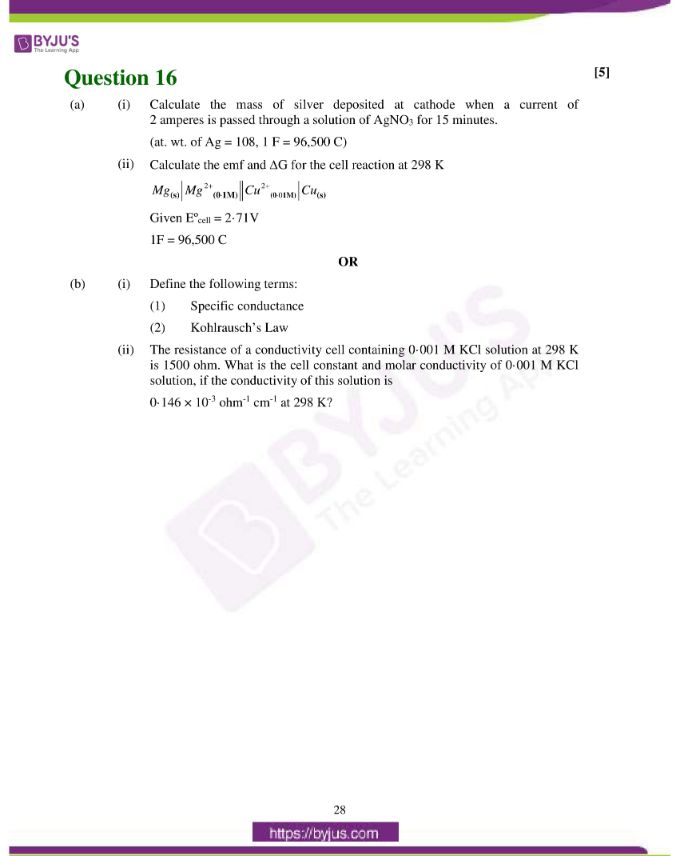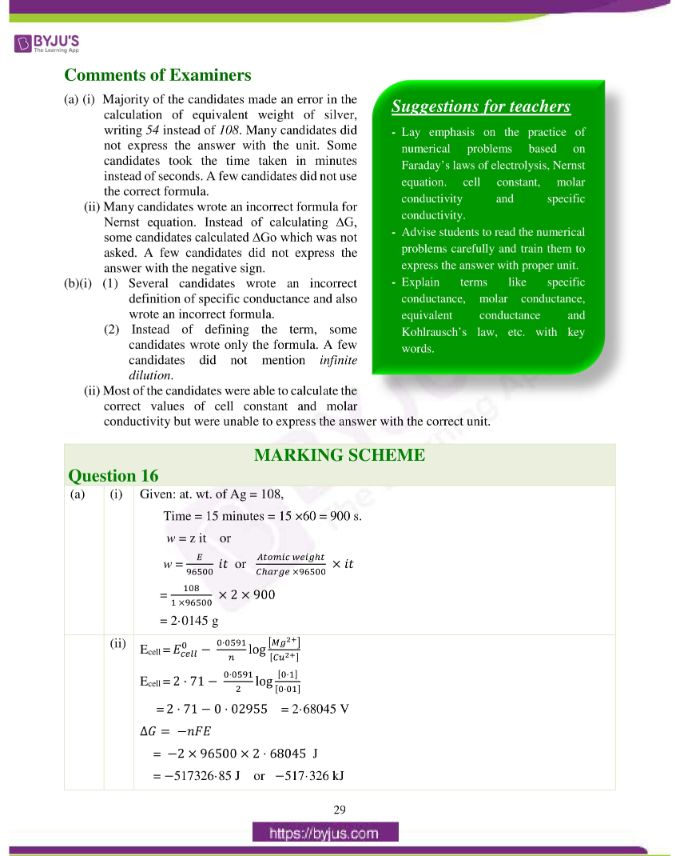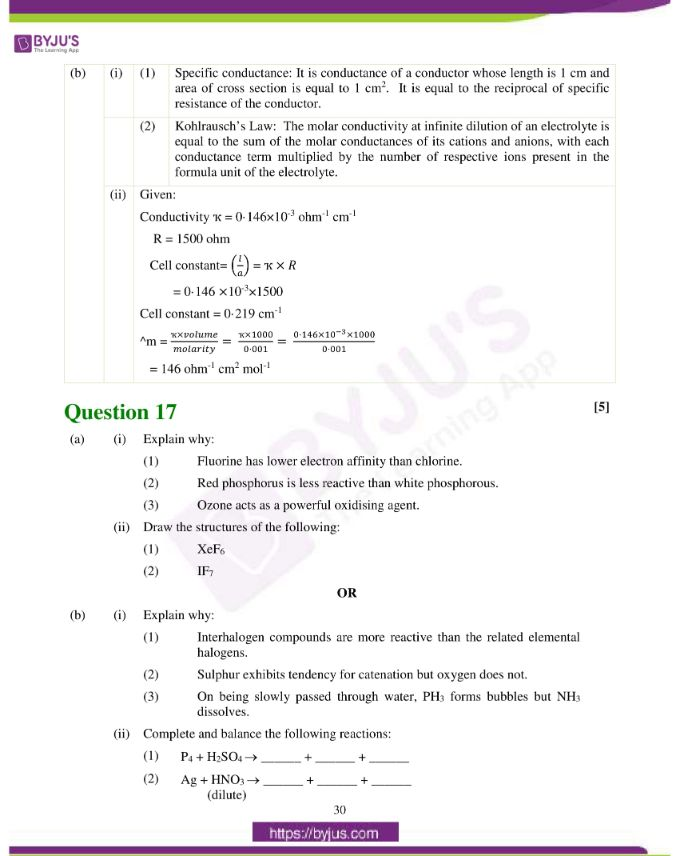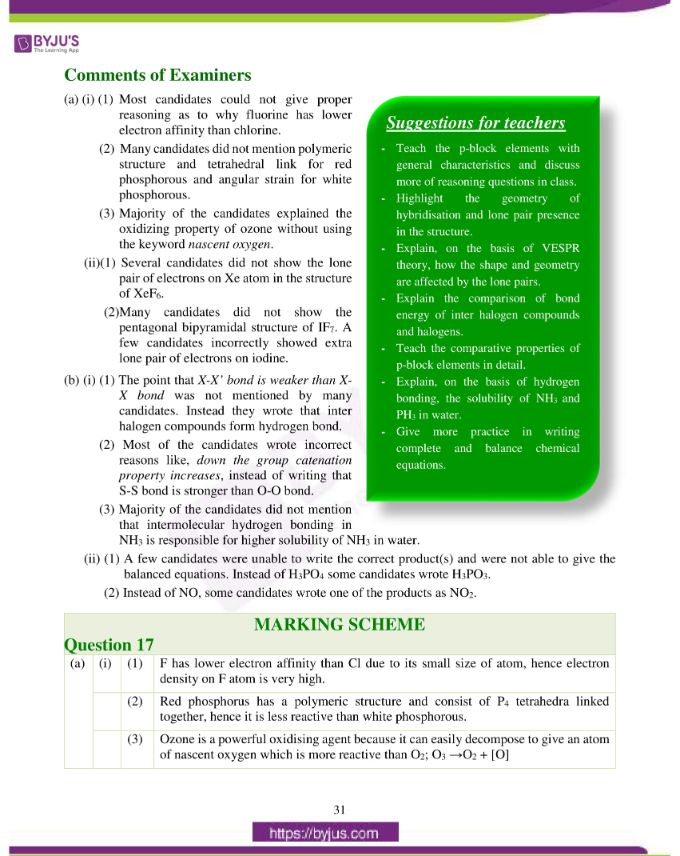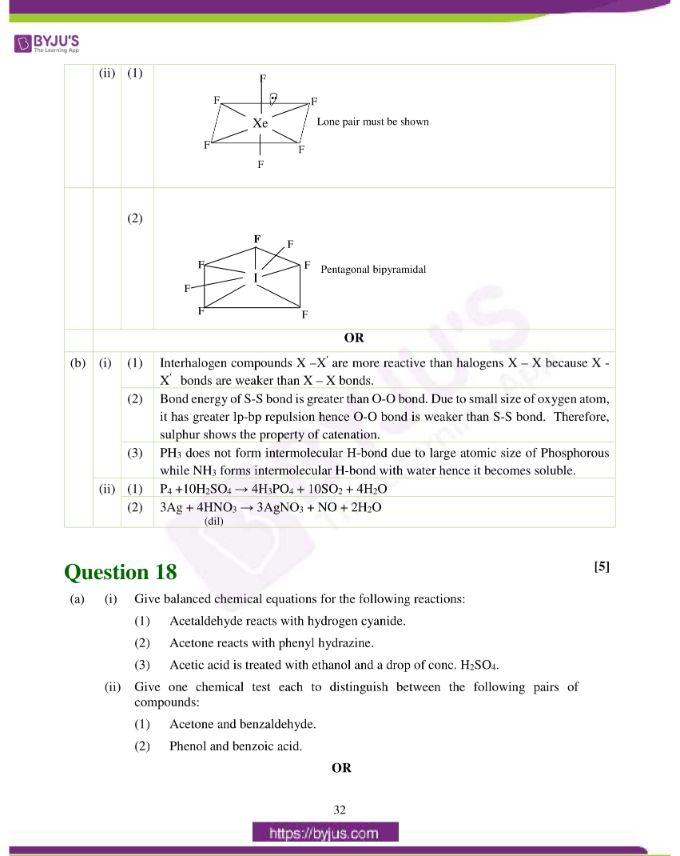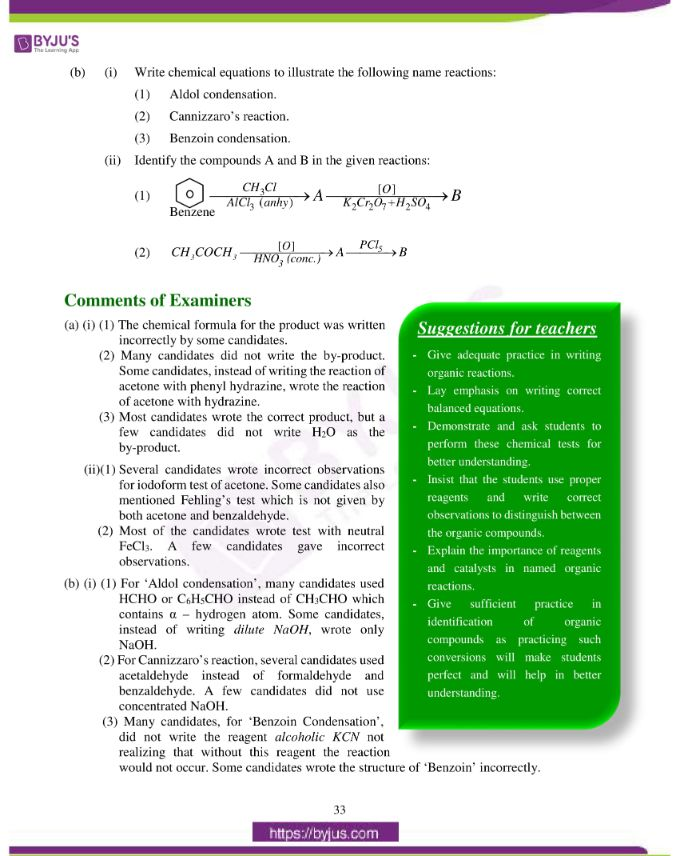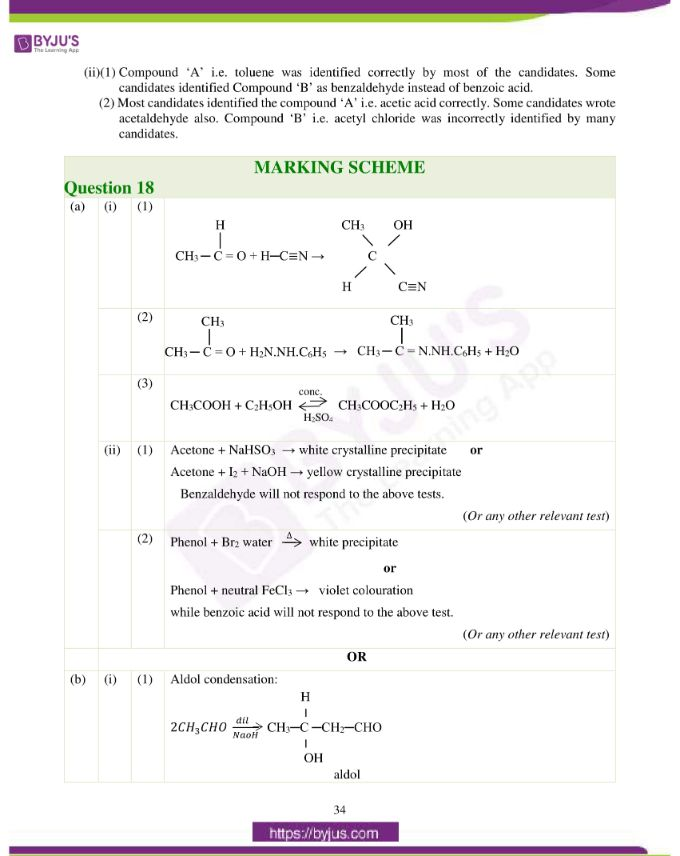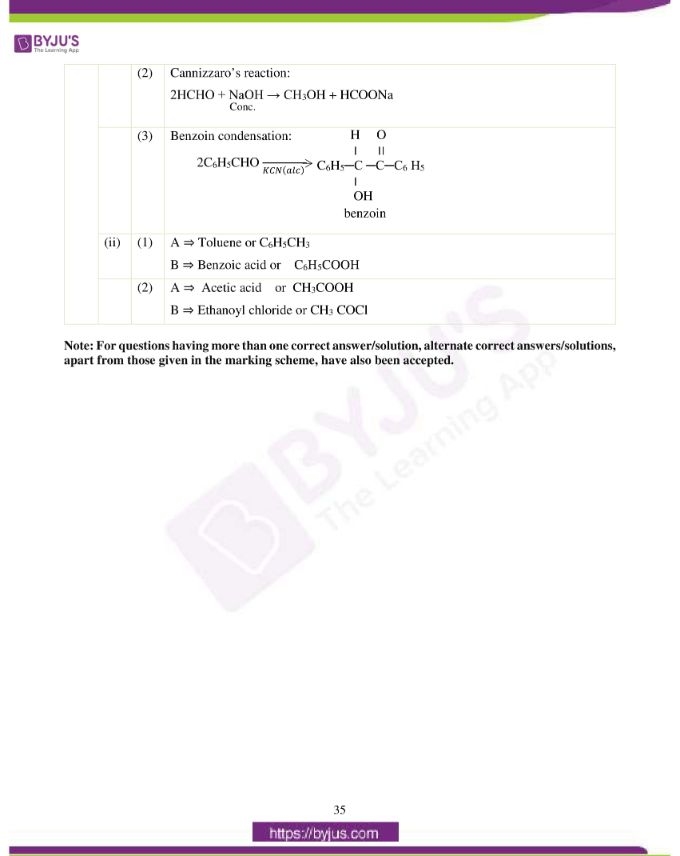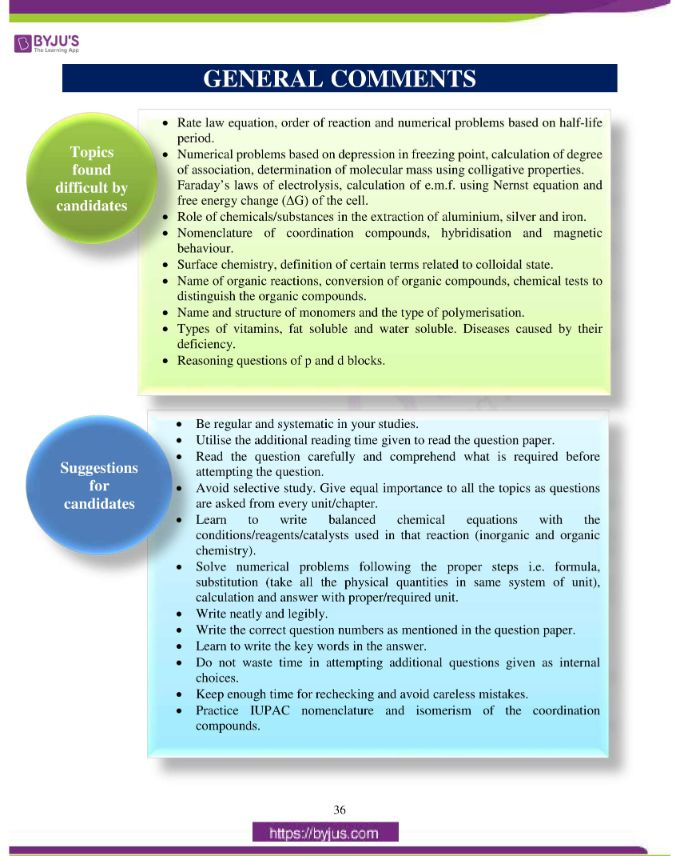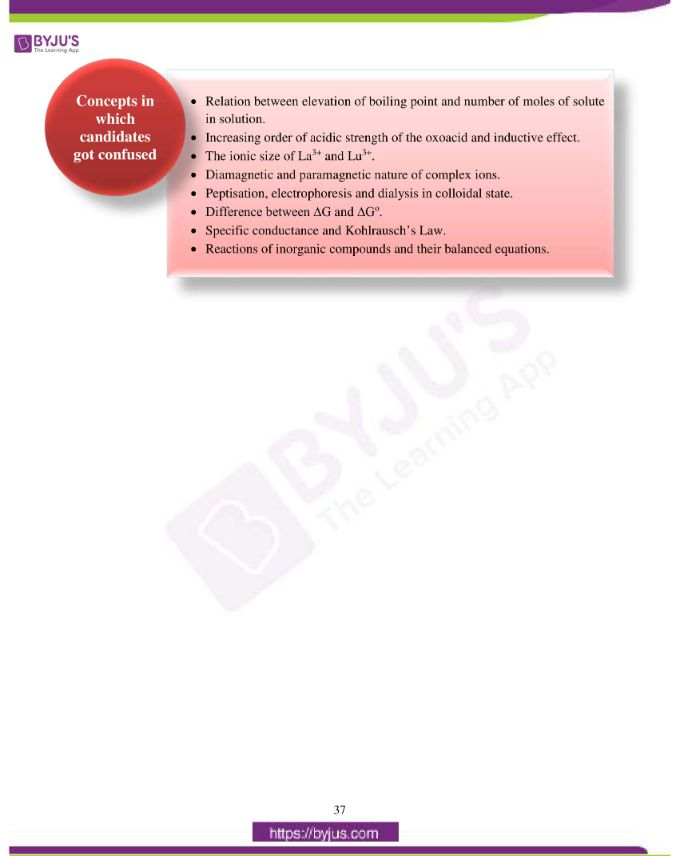### Difficult Topics of ISC Class 12 Chemistry Paper 2019

Topics which students found difficult while solving the Chemistry 2019 paper are listed below:

• Rate law equation, order of reaction and numerical problems based on half-life period.
• Numerical problems based on depression in freezing point, calculation of degree
• of association, determination of molecular mass using colligative properties. Faraday’s laws of electrolysis, calculation of e.m.f. using the Nernst equation and free energy change (ΔG) of the cell.
• Role of chemicals/substances in the extraction of aluminium, silver and iron.
• Nomenclature of coordination compounds, hybridisation and magnetic behaviour.
• Surface chemistry, definition of certain terms related to colloidal state.
• Name of organic reactions, conversion of organic compounds, chemical tests to distinguish the organic compounds.
• Name and structure of monomers and the type of polymerisation.
• Types of vitamins, fat soluble and water soluble. Diseases caused by their deficiency.
• Reasoning questions of p and d blocks.

### Confusing ISC Class 12 Chemistry Questions 2019

Chemistry concepts on which students got confused during the exam are mentioned below.

• Relation between elevation of boiling point and number of moles of solute in solution.
• Increasing order of acidic strength of the oxoacid and inductive effect.
• The ionic size of La3+ and Lu3+.
• Diamagnetic and paramagnetic nature of complex ions.
• Peptisation, electrophoresis and dialysis in colloidal state.
• Difference between ΔG and ΔGo.
• Specific conductance and Kohlrausch’s Law.
• Reactions of inorganic compounds and their balanced equations.

## ISC Class 12 Chemistry Question Paper Solution 2019

### Question 1:

(a) Fill in the blanks by choosing the appropriate word/words from those given in the brackets:

(more than, primary, cathode, Lucas reagent, two, four, less than, Grignard’s reagent, tertiary, anode, zero, equal to, three)

(i) The elevation of boiling point of 0·5 M K2SO4 solution is ___________that of 0·5 M urea solution. The elevation of boiling point of 0·5 M KCl solution is __________ that of 0·5 M K2SO4 solution.

(ii) A mixture of conc. HCl and anhydrous ZnCl2 is called __________ which shows maximum reactivity with _________ alcohol.

(iii) In electrolytic refining the impure metal is made __________ while a thin sheet of pure metal is used as _________.

(iv) When the concentration of a reactant of first order reaction is doubled, the rate of reaction becomes _________ times, but for a __________ order reaction, the rate of reaction remains the same.

(b) Select the correct alternative from the choices given:

(i) The cell reaction is spontaneous or feasible when emf of the cell is:

1. negative
2. positive
3. zero
4. either positive or negative

(ii) Which, among the following polymers, is a polyester:

1. melamine
2. bakelite
3. terylene
4. polythene

(iii) The correct order of increasing acidic strength of the oxoacids of chlorine is:

1. HClO3 < HClO4 < HClO2 < HClO
2. HClO < HClO2 < HClO3 < HClO4
3. HClO2 < HClO < HClO4 < HClO3
4. HClO3 < HClO4 < HClO < HClO2

(iv) A catalyst is a substance which:

1. changes the equilibrium constant of the reaction.
2. increases the equilibrium constant of the reaction.
3. supplies energy to the reaction.
4. shortens the time to reach equilibrium.

(c) Match the following:

 (i) Diazotisation (a) Anisotropic (ii) Crystalline solid (b) Reimer-Tiemann reaction (iii) Phenol (c) Diphenyl (iv) Fittig reaction (d) Aniline

(i)

1. Which trivalent ion has maximum size in the Lanthanide series i.e. Lanthanum ion (La3+) to Lutetium ion (Lu3+)? (at. no. of Lanthanum = 57 and Lutetium = 71)
2. Explain why Cu2+ is paramagnetic but Cu+ is diamagnetic. (at. no. of Cu = 29)

(ii) When a coordination compound CoCl3.6NH3 is mixed with AgNO3, three moles of AgCl are precipitated per mole of the compound. Write the structural formula and IUPAC name of the coordination compound.

(iii) Calculate the boiling point of urea solution when 6 g of urea is dissolved in 200 g of water.

(Kb for water = 0·52 K kg mol-1, boiling point of pure water = 373 K, mol. wt. of urea = 60)

(iv) Identify the compounds A, B, C and D in the given reaction: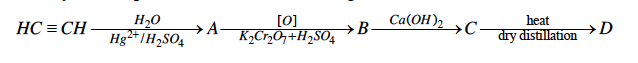(a) (i) More than, less than

(ii) Lucas reagent, tertiary

(iii) Anode, cathode

(iv) Two, zero

(b) (i) 2 or positive

(ii) 3 or terylene

(iii) 2 or HClO < HClO2 < HClO3 < HClO4

(iv) 4 or shortens the time to reach equilibrium

(c)

 (i) Diazotisation (d) Aniline (ii) Crystalline solid (a) Anisotropic (iii) Phenol (b) Reimer-Tiemann reaction (iv) Fittig reaction (c) Diphenyl

(d) (i)

1. La3+
2. Cu2+ is paramagnetic because it contains unpaired electrons in d orbital, Cu+ is diamagnetic as no unpaired electron is present.

(ii) [Co(NH3)6] Cl3

hexammine cobalt (III) chloride

(iii)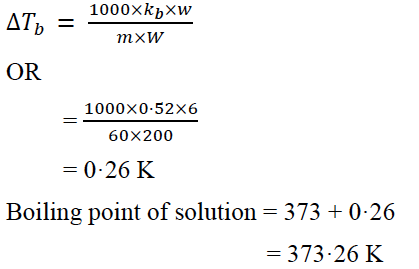(iv) A = CH3CHO

B = CH3COOH

C = (CH3COO)2Ca

D = CH3COCH3

### Question 2:

(a) For the reaction A + B → C + D, the initial rate for different reactions and initial concentration of reactants are given below:

 S. No. Initial Conc. Initial rate (mole L-1 sec-1) [A] mole L-1 [B] mole L-1 1 1.0 1.0 2 × 10 -3 2 2.0 1.0 4 × 10 -3 3 4.0 1.0 8 × 10 -3 4 1.0 2.0 2 × 10 -3 5 1.0 4.0 2 × 10 -3

(i) What is the overall order of reaction?

(ii) Write the rate law equation.

OR

(b) 25% of a first order reaction is completed in 30 minutes. Calculate the time taken in minutes for the reaction to go to 90% completion.

Answer: (a) (i) Overall order of reaction = 1 + 0 = 1

(ii) Rate Law equation= rate = k[A]1 [B]o

OR

rate = k[A]

(b)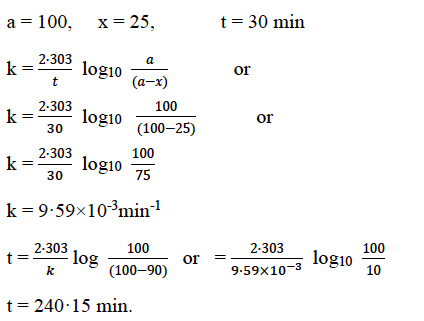### Question 3: (i) Name the type of drug which lowers the body temperature in high fever condition.(ii) What are tranquilizers? Give one example of a tranquilizer.

(ii) The chemical substances used for the treatment of stress, mild and severe mental diseases, anxiety and induce sleep are called tranquilizers.

Examples: Equanil, veronal, valium, etc.

### Question 4: Write the balanced chemical equation for each of the following:

(i) Chlorobenzene is treated with ammonia in the presence of Cu2O at 475 K and 60 atm.

(ii) Ethyl chloride treated with alcoholic potassium hydroxide.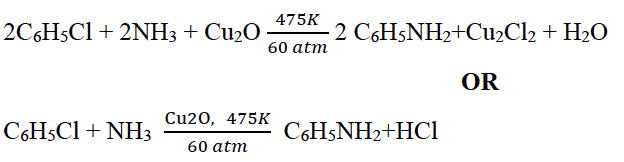(ii) CH3CH2Cl + KOH (alc) → CH2 = CH2 + KCl + H2O

### Question 6: Name two water soluble vitamins and the diseases caused by their deficiency in the diet of an individual.

Answer: Water soluble vitamins are B and C.

Diseases caused by deficiency of vitamin B are beriberi, dermatitis, pernicious anaemia, etc.

Disease caused by deficiency of vitamin C is Scurvy.

### Question 7:

(a) How will you obtain the following (give balanced chemical equations):

(i) Benzene from phenol.

(ii) Iodoform from ethanol.

OR

(b) How will you obtain the following (give balanced chemical equations):

(i) Salicylaldehyde from phenol.

(ii) Propan–2–ol from Grignard’s reagent.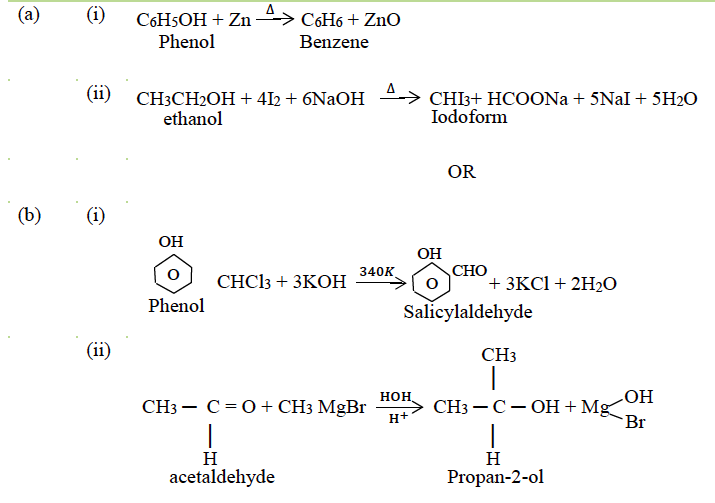### Question 8: Show that for a first order reaction the time required to complete 75% of reaction is about 2 times more than that required to complete 50% of the reaction.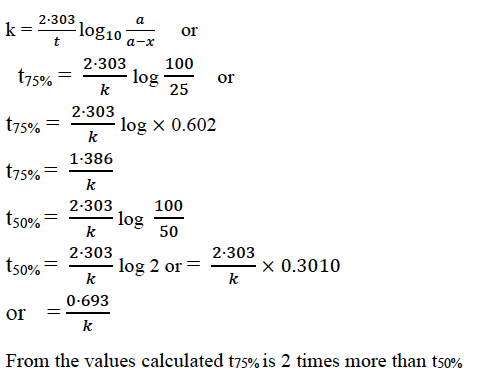### Question 9:

(a) When 0·4g of acetic acid is dissolved in 40g of benzene, the freezing point of the solution is lowered by 0·45K. Calculate the degree of association of acetic acid. Acetic acid forms dimer when dissolved in benzene.

(Kf for benzene = 5·12 K kg mol-1, at. wt. C = 12, H = 1, O = 16)

OR

(b) A solution is prepared by dissolving 9·25g of non-volatile solute in 450ml of water. It has an osmotic pressure of 350mm of Hg at 27ºC. Assuming the solute is non-electrolyte, determine its molecular mass.

(R = 0·0821 lit atm K-1 mol-1)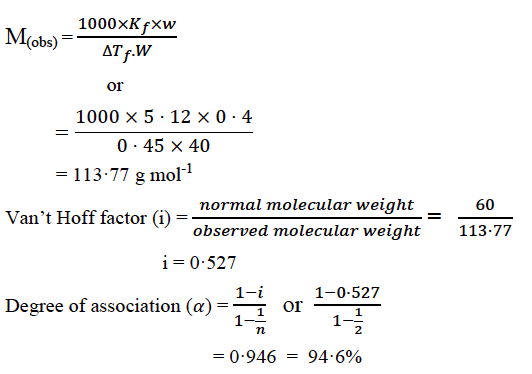(b)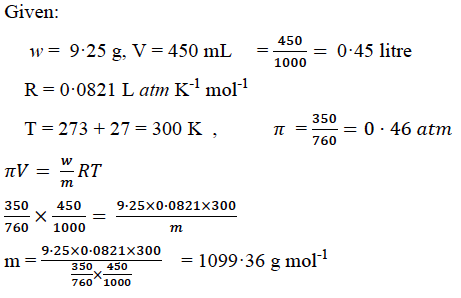### Question 10: An element occurs in body centered cubic structure. Its density is 8·0 g/cm3. If the cell edge is 250 pm, calculate the atomic mass of an atom of this element. (NA = 6·023 × 1023)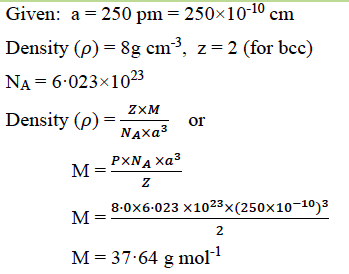### Question 11: Describe the role of the following:

(i) Cryolite in the extraction of aluminium from pure alumina.

(ii) NaCN in the extraction of silver from a silver ore.

(iii) Coke in the extraction of iron from its oxides.

Answer: (i) In order to decrease the melting point of mixture and to increase the conductivity of alumina (Al2O3), cryolite is added in the extraction of aluminium.

(ii) When silver ore is treated with NaCN solution, silver ore gets dissolved and forms a soluble complex whereas impurities remain insoluble.

(iii) Coke reduces CO2 to CO which helps in the reduction of iron oxide to iron.

Or CO2 (g) + C (s) → 2CO(g)

Fe2O3 + 3CO → 2Fe + 3CO2

### Question 12:

(i) Write the IUPAC names of the following:

1. K3[Fe(C2O4)3]
2. [Co(NH3)5Cl]SO4

(ii) [Fe(CN)6]4- is a coordination complex ion.

1. Calculate the oxidation number of iron in the complex.
2. Is the complex ion diamagnetic or paramagnetic?
3. What is the hybridisation state of the central metal atom?
4. Write the IUPAC name of the complex ion.

1. Potassiumtrioxalatoferrate (III)
2. Pentaamminechloridocobalt (III) sulphate

(ii)

1. +2
2. Diamagnetic
3. d2 sp3
4. Hexacyanoferrate (II) ion

### Question 13:

(a) Explain why:

(i) Transition elements form alloys.

(ii) Zn2+ salts are white whereas Cu2+ salts are coloured.

(iii) Transition metals and their compounds act as catalyst.

OR

(b) Complete and balance the following chemical equations.

(i) KMnO4 + H2SO4 + H2C2O4 → ______ + ______ + ______ + ______

(ii) K2Cr2O7 + H2SO4 + KI → ______ + ______ + ______ + ______

(iii) K2Cr2O7 + H2SO4 + FeSO4 → ______ + ______ + ______ + ______

(i) The atomic radii of transition elements in any series are not much different and hence can replace each other easily in lattice.

(ii) Zn2+ does not have any unpaired electron while Cu2+ has an unpaired electron and can undergo d-d transition emitting colour.

(iii) Transition elements have variable oxidation state giving unstable intermediates, thus act as catalysts.

OR

(i) KMnO4 + H2SO4 + H2C2O4 → K2SO4 + 2MnSO4 + 8H2O + 10CO2

(ii) K2Cr2O7 + H2SO4 + KI → 4K2SO4 + Cr2(SO4)3+ 7H2O + 3I2

(iii) K2Cr2O7 + H2SO4 + FeSO4 → Fe2(SO4)3 + K2SO4 + Cr2(SO4)3 + 7H2O

### Question 14: Give balanced equations for the following:

(i) Aniline is treated with bromine water.

(ii) Ethylamine is heated with chloroform and alcoholic solution of potassium hydroxide.

(iii) Benzene diazonium chloride is treated with ice cold solution of aniline in acidic medium.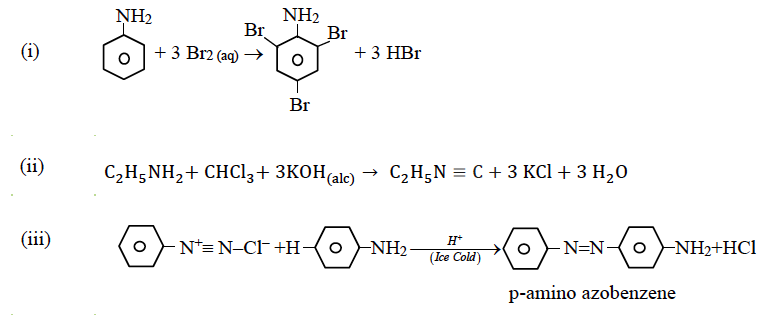### Question 15: Define the following terms with suitable examples:

(i) Peptisation

(ii) Electrophoresis

(iii) Dialysis

Answer: (i) Peptisation: The process of conversion of a fresh precipitate into colloidal solution by shaking it with dispersion medium in the presence of electrolyte is called peptisation.

Example: Red coloured solution of Fe(OH)3 is obtained when freshly prepared precipitate is treated with a small amount of FeCl3 solution.

(ii) Electrophoresis: The movement of colloidal particles under an applied electric potential is called electrophoresis. Positively charged particles move towards cathode, while negatively charged particles move towards the anode.

Example: purification of sewage water, proteins, etc.

(iii) Dialysis: It is the process of removing a dissolved substance from a colloidal solution by means of diffusion through a suitable membrane. The molecules or ions diffuse through the membrane and a pure colloidal solution is left behind.

Example: removal of sugar from blood in diabetic patients.

### Question 16:

(a) (i) Calculate the mass of silver deposited at cathode when a current of 2 amperes is passed through a solution of AgNO3 for 15 minutes. (at. wt. of Ag = 108, 1 F = 96,500 C)

(ii) Calculate the emf and G for the cell reaction at 298 K

Mg(s) | Mg2+(0.1M) || Cu2+(0.01M) | Cu(s)

Given Eºcell = 2·71V

1F = 96,500 C

(b) (i) Define the following terms:

1. Specific conductance
2. Kohlrausch’s Law

(ii) The resistance of a conductivity cell containing 0·001 M KCl solution at 298 K is 1500 ohm. What is the cell constant and molar conductivity of 0·001 M KCl solution, if the conductivity of this solution is 0·146 × 10-3 ohm-1 cm-1 at 298 K?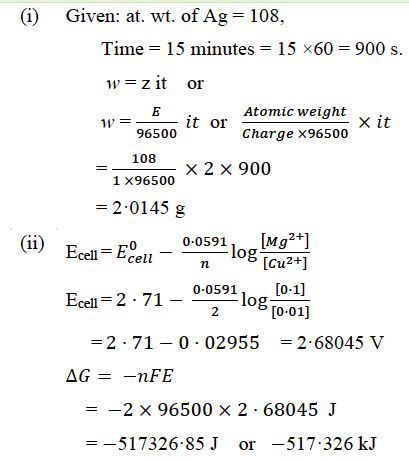(b) (i)

1. Specific conductance: It is conductance of a conductor whose length is 1 cm and area of cross section is equal to 1 cm2. It is equal to the reciprocal of specific resistance of the conductor.
2. Kohlrausch’s Law: The molar conductivity at infinite dilution of an electrolyte is equal to the sum of the molar conductances of its cations and anions, with each conductance term multiplied by the number of respective ions present in the formula unit of the electrolyte.

(ii)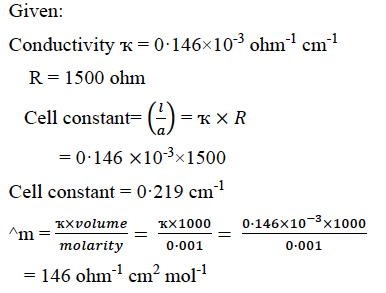### Question 17:

(a) (i) Explain why:

1. Fluorine has lower electron affinity than chlorine.
2. Red phosphorus is less reactive than white phosphorus.
3. Ozone acts as a powerful oxidising agent.

(ii) Draw the structures of the following:

1. XeF6
2. IF7

OR

(b) (i) Explain why:

1. Interhalogen compounds are more reactive than the related elemental halogens.
2. Sulphur exhibits a tendency for catenation but oxygen does not.
3. On being slowly passed through water, PH3 forms bubbles but NH3 dissolves.

(ii) Complete and balance the following reactions:

1. P4 + H2SO4 → ______ + ______ + ______
2. Ag + HNO3 (dilute) → ______ + ______ + ______

1. F has lower electron affinity than Cl due to its small size of atom, hence electron density on F atom is very high.
2. Red phosphorus has a polymeric structure and consists of P4 tetrahedra linked together, hence it is less reactive than white phosphorus.
3. Ozone is a powerful oxidising agent because it can easily decompose to give an atom of nascent oxygen which is more reactive than O2; O3 → O2 + [O]

(ii) (1)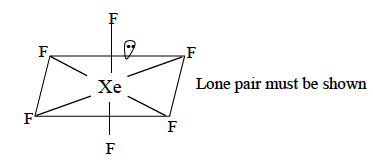(2)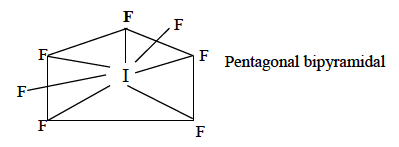(b) (i)

1. Interhalogen compounds X –Xʹ are more reactive than halogens X – X because X – Xʹ bonds are weaker than X – X bonds.
2. Bond energy of S-S bond is greater than O-O bond. Due to the small size of oxygen atom, it has greater lp-bp repulsion hence O-O bond is weaker than S-S bond. Therefore, sulphur shows the property of catenation.
3. PH3 does not form intermolecular H-bonds due to large atomic size of Phosphorous while NH3 forms intermolecular H-bonds with water hence it becomes soluble.

(ii)

1. P4 +10H2SO4 → 4H3PO4 + 10SO2 + 4H2O
2. 3Ag + 4HNO3(dil) → 3AgNO3 + NO + 2H2O

### Question 18:

(a) (i) Give balanced chemical equations for the following reactions:

1. Acetaldehyde reacts with hydrogen cyanide.
2. Acetone reacts with phenyl hydrazine.
3. Acetic acid is treated with ethanol and a drop of conc. H2SO4.

(ii) Give one chemical test each to distinguish between the following pairs of compounds:

1. Acetone and benzaldehyde.
2. Phenol and benzoic acid.

OR

(b) (i) Write chemical equations to illustrate the following name reactions:

1. Aldol condensation.
2. Cannizzaro’s reaction.
3. Benzoin condensation.

(ii) Identify the compounds A and B in the given reactions: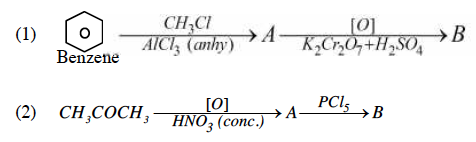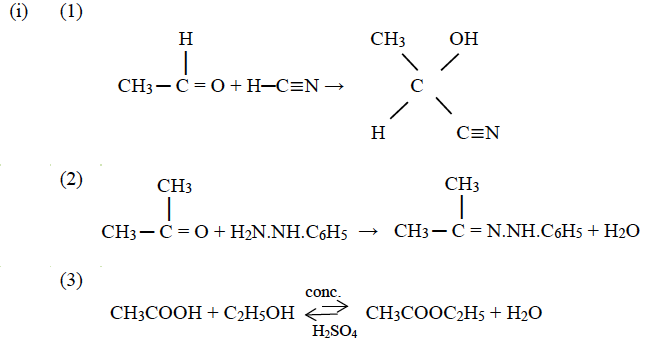(ii)

1. Acetone + NaHSO3 → white crystalline precipitate or

Acetone + I2 + NaOH → yellow crystalline precipitate

Benzaldehyde will not respond to the above tests.

1. Phenol + Br2 water Δ white precipitate

OR

Phenol + neutral FeCl3 → violet colouration

while benzoic acid will not respond to the above test.

(b) (i) (1)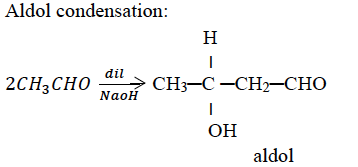(2) Cannizzaro’s reaction:

2HCHO + NaOH (Conc.) → CH3OH + HCOONa

(3)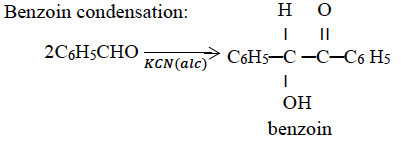(ii)

1. A ⇒ Toluene or C6H5CH3

B ⇒ Benzoic acid or C6H5COOH

1. A ⇒ Acetic acid or CH3COOH
2. B ⇒ Ethanoyl chloride or CH3COCl

The ISC Class 12 Chemistry Question Paper Solution 2019 must have helped students with their exam preparation. Apart from the 2019 paper solution, students can also find the answers to other papers of ISC Class 12 Previous Years Questions by clicking here. Happy Learning and stay tuned to BYJU’S for the latest update on ICSE/CBSE/State Boards/Competitive exams. Also, don’t forget to download the BYJU’S App.